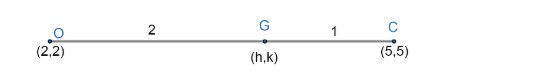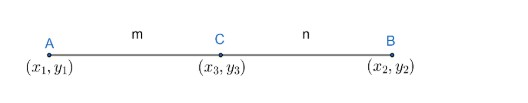Courses
Courses for Kids
Free study material
Offline Centres
MoreLast updated date: 04th Dec 2023
Total views: 383.1k
Views today: 7.83k

# If coordinates of the circumcentre and the orthocentre of a triangle are respectively (5,5) and (2,2), then the coordinates of the centroid are(A) (1,1) (B) (3,1) (C) (3,3) (D) (2,2) (E) (4,4)Verified
383.1k+ views
Hint: Use the relation among centroid, circumcentre and orthocentre. Centroid is the point which divides the line joining orthocentre to circumcentre. Use the section formula to get centroid.

Here, we have given coordinates of circumcentre and orthocentre and we have to find coordinates of centroid. So, we need to use some relationship between all three of them.
As we know that centroid divides line joining orthocentre and circumcentre into 2: 1 ratio.
We have coordinates of
Orthocentre = (2,2)
Circumcentre = (5,5)
Let the coordinates of the centroid is (h,k).
So, we can represent the above now as;Here, we have to find coordinates of G, which divides OC in 2: 1. So, we need to use sectional formulas to get coordinates of G.
Sectional formula is defined as if two points $A\left( {{x}_{1}},{{y}_{1}} \right)\text{ and }B\left( {{x}_{2}},{{y}_{2}} \right)$ are given on a line and any third point $C\left( {{x}_{3}},{{y}_{3}} \right)$ on the same line divides AB in ration m: n, then we can write point C as;\begin{align} & {{x}_{3}}=\dfrac{m{{x}_{2}}+n{{x}_{1}}}{m+n} \\ & {{y}_{3}}=\dfrac{m{{y}_{2}}+n{{y}_{1}}}{m+n} \\ \end{align}
Hence, coordinates of point C is $\left( \dfrac{m{{x}_{2}}+n{{x}_{1}}}{m+n},\dfrac{m{{y}_{2}}+n{{y}_{1}}}{m+n} \right)$
Now, coming to the question, we can use the sectional formula to get the centroid and where ratio m: n is given as (2: 1).
Therefore, we can use sectional formula to get G.
\begin{align} & G\left( h,k \right)=\left( \dfrac{2\times 5+1\times 2}{2+1},\dfrac{2\times 5+1\times 2}{2+1} \right) \\ & G\left( h,k \right)=\left( \dfrac{12}{3},\dfrac{12}{3} \right)=\left( 4,4 \right) \\ \end{align}
Hence, coordinates of centroid id (4,4) if orthocentre and circumcentre are (2,2) and (5,5).
Option (E) is the correct option.
Note: One can go wrong while applying sectional formula. As one can apply formula as $\left( \dfrac{m{{x}_{1}}+n{{x}_{2}}}{m+n},\dfrac{m{{y}_{1}}+n{{y}_{2}}}{m+n} \right)$ which is wrong, so terms in sectional formula is very important.
One can take a triangle with coordinates as $\left( {{x}_{1}},{{y}_{1}} \right),\left( {{x}_{2}},{{y}_{2}} \right)\And \left( {{x}_{3}},{{y}_{3}} \right)$ and try to calculate $\left( {{x}_{1}},{{y}_{1}} \right),\left( {{x}_{2}},{{y}_{2}} \right)\And \left( {{x}_{3}},{{y}_{3}} \right)$ with the help of given orthocentre and circumcentre. But it will be a very long process and is not necessary for this question. So, we need to use the concept of relation between orthocentre, circumcentre and centroid. Centroid divides orthocentre and circumcentre in 2: 1 part.